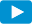Notes for Level I CFA® Program

R54 Understanding Fixed-Income Risk and Return

3.5. Duration of a Bond PortfolioWatch VideoIn the previous section, we saw how to calculate the duration for an individual bond. What if a portfolio consists of a number of bonds: how will its duration be calculated?

There are two ways to calculate the duration of a bond portfolio:

• Weighted average of time to receipt of the aggregate cash flows.
• Weighted average of the individual bond durations that comprise the portfolio.

The key points related to each method are outlined in the table below.

 Weighted Average Time to Receipt of Aggregate Cash Flows Weighted Average of Individual Bond Durations in Portfolio Theoretically correct, but difficult to use in practice. Commonly used in practice. Cash flow yield not commonly used. Cash flow yield is the IRR on a series of cash flows. Easy to use as a measure of interest rate risk. Amount and timing of cash flows might not be known because some of these bonds may be MBS, or with call options. More accurate as difference in YTMs of bonds in portfolio become smaller. Interest rate risk is usually expressed as a change in benchmark interest rates, not as a change in the cash flow yield. Assumes parallel shifts in the yield curve, i.e. all rates change by the same amount in the same direction that seldom happens in reality. Change in the cash flow yield is not necessarily the same amount as the change in yields-to-maturity on the individual bonds.

Example 12: Calculating portfolio duration

An investment fund owns the following portfolio of three fixed-rate government bonds:

 Bond A Bond B Bond C Par value 15,000,000 20,000,000 40,000,000 Market value 14,769,542 25,650,379 43,796,854 Modified duration 4.328 5.643 7.210

The total market value of the portfolio is 84,216,775. Each bond is on a coupon date so that there is no accrued interest. The market values are the full prices given the par value.

1. Calculate the average modified duration for the portfolio using the shares of market value as the weights.
2. Estimate the percentage loss in the portfolio’s market value if the yield-to-maturity on each bond goes up by 10 bps.

Solution to 1:

The average modified duration of the portfolio is 6.23.

(14,769,542/84,216,775) × 4.328 + (25,650,379/84,216,775) × 5.643 + (43,796,854/84,216,775) × 7.210 = 6.23.

Solution to 2:

The estimated decline in market value if each yield rises by 10 bps is 0.623%. -6.23 × 0.001 = -0.00623.